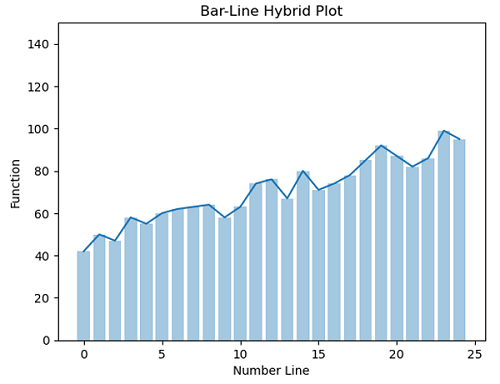# Python | Bar-Line Hybrid Plot

Bar-Line Hybrid Plot in Python. Here, we are going to learn about the Bar-Line Hybrid Plot and its Python implementation.
Submitted by Anuj Singh, on July 18, 2020

In some of the cases, we need to plot a bar-line hybrid plot. This plot helps in a better understanding of dynamics as well as the relative magnitude of each point in the plot. Bar-Line Hybrid Plots are mostly used for the representation of smaller data sets.

The following example illustrate the implementation and use of the Bar-Line Hybrid Plot.## Python code for bar-line hybrid plot

```import numpy as np
import matplotlib.pyplot as plt

x = np.arange(25)
y1 = np.arange(25)
for i in range(25):
y1[i] = 2*x[i] + np.random.randint(40,56)

plt.figure()
plt.plot(x,y1)
plt.bar(x,y1, alpha=0.4)
plt.ylim(0,150)
plt.xlabel('Number Line')
plt.ylabel('Function')
plt.title('Bar-Line Hybrid Plot')
```

Output:

```Output is as figure
```

Languages: » C » C++ » C++ STL » Java » Data Structure » C#.Net » Android » Kotlin » SQL
Web Technologies: » PHP » Python » JavaScript » CSS » Ajax » Node.js » Web programming/HTML
Solved programs: » C » C++ » DS » Java » C#
Aptitude que. & ans.: » C » C++ » Java » DBMS
Interview que. & ans.: » C » Embedded C » Java » SEO » HR
CS Subjects: » CS Basics » O.S. » Networks » DBMS » Embedded Systems » Cloud Computing
» Machine learning » CS Organizations » Linux » DOS
More: » Articles » Puzzles » News/Updates